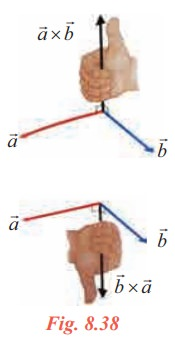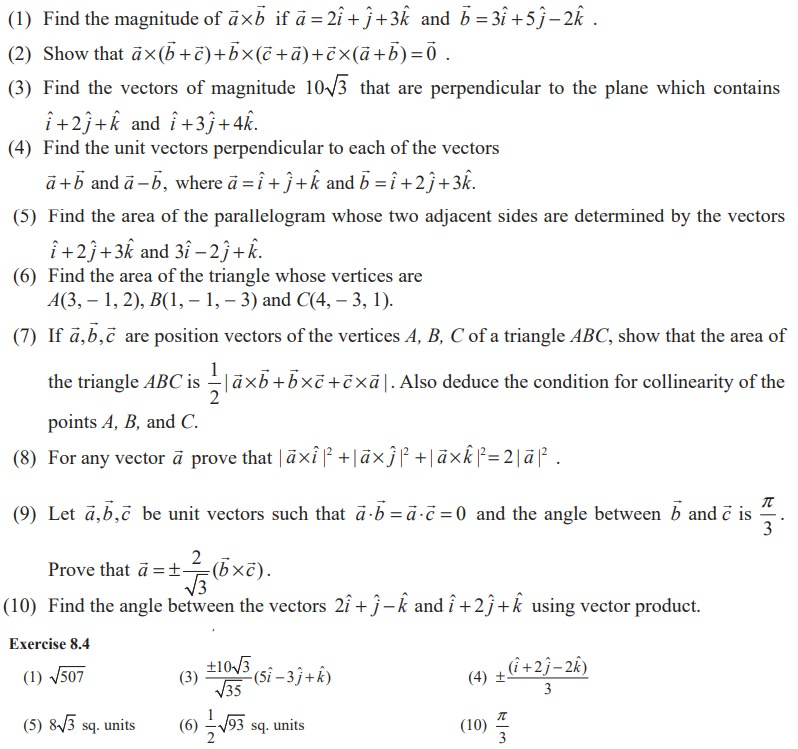Home | | Maths 11th std | Vector Product and Properties of Vector Product

# Vector Product and Properties of Vector Product

To define vector product between two vectors, we need the concept of right handed and left handed system.

Vector Product

To define vector product between two vectors, we need the concept of right handed and left handed system.

If we align the fingers of our right hand along the vectorand bend our fingers around in the direction of rotation fromtowards(through an angle  less than 180º ), our thumb will point in the direction of×. Now, following the right-hand rule,×will point in the direction opposite to×(See Fig. 8.38).We may also say that ifis rotated into the direction ofthrough the angle θ(<π) , then×advances in the same direction as a right-handed screw would if turned in the same way.A Cartesian coordinate system is called right-handed if the corresponding unit vectors iˆ, ˆj , kˆ in the positive direction of the axes form a right-handed triple as in Fig. 8.39. The system is called left handed if the sense of kˆ is reversed as in Fig 8.40.

Definition 8.17

Vector product of any two non-zero vectorsandis written as×and is defined asThe resultant is a vector with magnitude |||| sinθ and has the direction nˆ.

Furtherxis a vector perpendicular to bothand. That is,xis normal to the plane containingand.

Note 8.6

(i) Note that the order of the vectors is very important to decide the direction of nˆ .

(ii) Since the resultant is a vector, this product is named as vector product. Again, we use the symbol cross ‘×’ to define such a product and hence it has another name cross product.

Geometrical interpretation of vector product

Construct a parallelogram OACB with=and=as adjacent sides.

Let ∟AOB = θThus,×is a vector whose magnitude is the area of the parallelogram, havingandas its adjacent sides and whose direction nˆ is perpendicular to the plane containingandsuch that,, nˆ form a right handed system.

Thus, |×| = area of the parallelogram whose adjacent sides areand.

From the area of the parallelogram, we can deduct the area of the triangle OAC as half of the area of OACB.

Deduction

The area of any triangle whose two sides areand= 1/2 |×|Properties

(i)  Vector product is non-commutative

By definitionThus vector product is non-commutative.

(ii) If two vectors are collinear or parallel then×= 0 (how?)

But or or×= 0 ⇒= 0= 0andare parallel.

(iii) For any two non-zero vectorsand b ,× b =andare parallel.

Deductionx=(iv) With usual meaning of iˆ , jˆ and kˆ (they form a right handed system), the following results are obtained.It is clear that,

iˆ × iˆ = jˆ × jˆ = kˆ × kˆ =iˆ × jˆ = kˆ

jˆ × kˆ = iˆ ;

kˆ × iˆ = jˆ

jˆ × iˆ = −kˆ ;

kˆ × jˆ = −iˆ

iˆ × kˆ = − jˆ (how?)Note 8.7

In this case θ is always acute. Thus, if we try to find the angle using vector product, we get only the acute angle. Hence in problems of finding the angle, the use of dot product is preferable since it specifies the position of the angle θ .

(x) The unit vectors perpendicular to bothandareVectors of magnitude λ, perpendicular to bothandareNote 8.8

Instead ofand, one can take any two sides.

EXERCISE 8.4Study Material, Lecturing Notes, Assignment, Reference, Wiki description explanation, brief detail
11th Mathematics : UNIT 8 : Vector Algebra I : Vector Product and Properties of Vector Product |